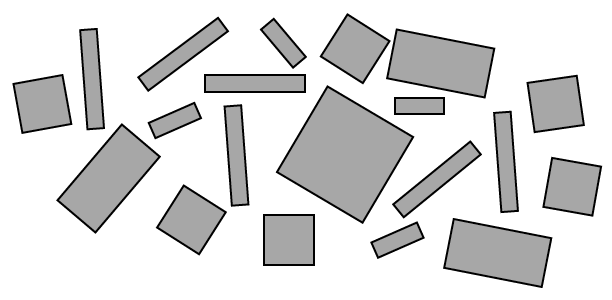### Home > INT1 > Chapter A > Lesson A.1.1 > ProblemA-7

A-7.

Write a simplified algebraic expression for the tile collection below. (The names of the algebra tiles can be found in problem A-1.)To solve this problem, you will need to group the tiles by their areas.
How many $x$-tiles are there? $x^{2}$-tiles?

$6\textit{x}^2+4\textit{x}+3\textit{xy}+6\textit{y}+\textit{y}^2$

Use the eTool below to write a simplified expression.
Click the link at the right to view full version of the eTool: A-7 HW eTool